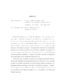## Numerical studies of constraints and gravitational wave extraction in general relativity

 dc.contributor.advisor Misner, Charles W en_US dc.contributor.author Fiske, David Robert en_US dc.contributor.department Physics en_US dc.contributor.publisher Digital Repository at the University of Maryland en_US dc.contributor.publisher University of Maryland (College Park, Md.) en_US dc.date.accessioned 2004-08-27T05:34:21Z dc.date.available 2004-08-27T05:34:21Z dc.date.issued 2004-08-04 en_US dc.description.abstract Within classical physics, general relativity is the theory of gravity. Its equations are non-linear partial differential equations for which relatively few closed form solutions are known. Because of the growing observational need for solutions representing gravitational waves from astrophysically plausible sources, a subfield of general relativity, numerical relativity, has a emerged with the goal of generating numerical solutions to the Einstein equations. This dissertation focuses on two fundamental problems in modern numerical relativity: (1) Creating a theoretical treatment of the constraints in the presence of constraint-violating numerical errors, and (2) Designing and implementing an algorithm to compute the spherical harmonic decomposition of radiation quantities for comparison with observation. On the issue of the constraints, I present a novel and generic procedure for incorporating the constraints into the equations of motion of the theory in a way designed to make the constraint hypersurface an attractor of the evolution. In principle, the prescription generates non-linear corrections for the Einstein equations. The dissertation presents numerical evidence that the correction terms do work in the case of two formulations of the Maxwell equations and two formulations of the linearized Einstein equations. On the issue of radiation extraction, I provide the first in-depth analysis of a novel algorithm, due originally to Misner, for computing spherical harmonic components on a cubic grid. I compute explicitly how the truncation error in the algorithm depends on its various parameters, and I also provide a detailed analysis showing how to implement the method on grids in which explicit symmetries are enforced via boundary conditions. Finally, I verify these error estimates and symmetry arguments with a numerical study using a solution of the linearized Einstein equations known as a Teukolsky wave. The algorithm performs well and the estimates prove true both in simulations run on a uniform grid and in simulations that make use of fixed mesh refinement techniques. en_US dc.format.extent 905503 bytes dc.format.mimetype application/pdf dc.identifier.uri http://hdl.handle.net/1903/1805 dc.language.iso en_US dc.subject.pqcontrolled Physics, Astronomy and Astrophysics en_US dc.subject.pqcontrolled Mathematics en_US dc.subject.pqcontrolled Computer Science en_US dc.subject.pquncontrolled general relativity en_US dc.subject.pquncontrolled constraints en_US dc.subject.pquncontrolled gravitational wave en_US dc.subject.pquncontrolled spherical harmonics en_US dc.subject.pquncontrolled partial differential equations en_US dc.subject.pquncontrolled computer simulation en_US dc.title Numerical studies of constraints and gravitational wave extraction in general relativity en_US dc.type Dissertation en_US
##### Original bundle
Now showing 1 - 1 of 1